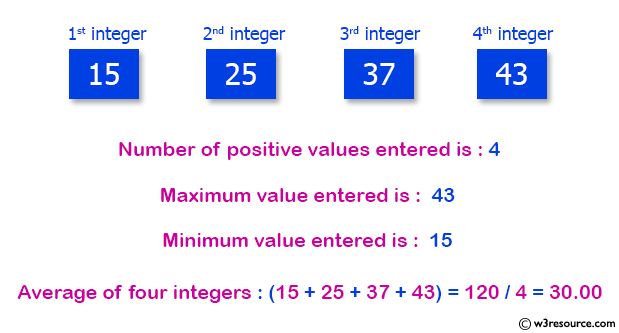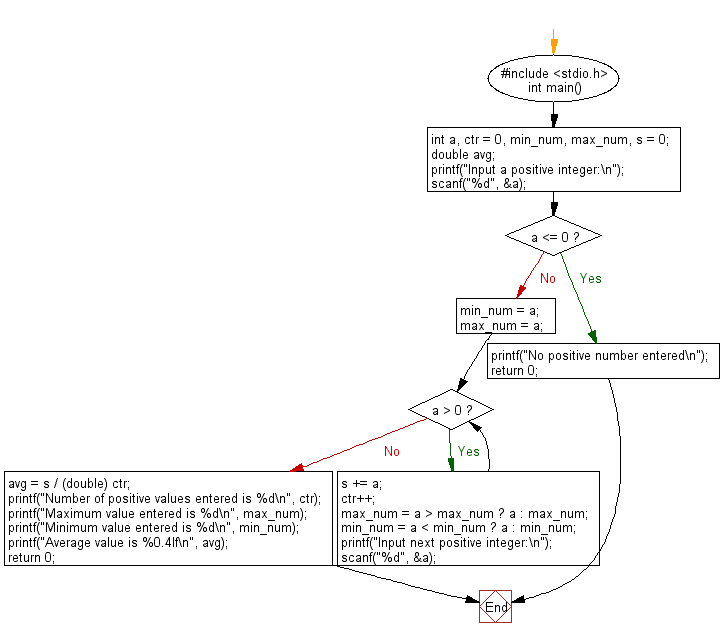﻿ C : Positive, minimum, maximum, average of all numbers

# C Exercises: Display the number of positive values, the minimum value, the maximum value and the average of all numbers

## C Basic Declarations and Expressions: Exercise-64 with Solution

Write a C program that accepts integers from the user until a zero or a negative number, displays the number of positive values, the minimum value, the maximum value, and the average value.

Test data and expected output:
5 2 5 8 9 12 0

Pictorial Presentation:Sample Solution:

C Code:

``````#include <stdio.h>
int main() {
int a, ctr = 0, min_num, max_num, s = 0;
double avg;

// Prompt user for input
printf("Input a positive integer:\n");

// Read the first input value
scanf("%d", &a);

// Check if the input is positive
if (a <= 0) {
printf("No positive number entered\n");
return 0;
}

// Initialize min_num and max_num with the first input value
min_num = a;
max_num = a;

// Loop to process subsequent inputs
while (a > 0) {
s += a;
ctr++;
max_num = a > max_num ? a : max_num;
min_num = a < min_num ? a : min_num;

// Prompt for the next positive integer
printf("Input next positive integer:\n");
scanf("%d", &a);
}

avg = s / (double) ctr;

// Display the results
printf("Number of positive values entered is %d\n", ctr);
printf("Maximum value entered is %d\n", max_num);
printf("Minimum value entered is %d\n", min_num);
printf("Average value is %0.4lf\n", avg);

return 0;
}
``````

Sample Output:

```Input a positive integer:
Input next positive integer: 15
Input next positive integer: 25
Input next positive integer: 37
Input next positive integer: 43
Number of positive values entered is 4
Maximum value entered is 43
Minimum value entered is 15
Average value is 30.0000
```

Flowchart:C programming Code Editor:

What is the difficulty level of this exercise?

Test your Programming skills with w3resource's quiz.

﻿